TUTTI Jaime is 3 years older than Crystal. Marissa is twice as old Jaime. The sum of their ages is 45. How old is each

Question

TUTTI
Jaime is 3 years older than Crystal.
Marissa is twice as old Jaime. The sum
of their ages is 45. How old is each
person?
a) Define a variable:
b) Write an equation.
c) Solve the equation.
complete sentence.

in progress 0
6 months 2021-08-10T20:19:19+00:00 1 Answers 4 views 0

See Explanation

Step-by-step explanation:

Solving (a): The Variables

The variables are the ages of Jaime, Crystal and Marissa;

Let

x represents Jaime’s age;

y represents Crystal’s age;

z represents Marissa’s age

Solving (b): Equation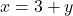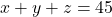Solving (c): Result of the equations— (1)— (2)— (3)

Make y the subject in (1)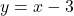Substituteandin (3)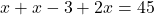Collect Like Terms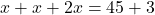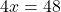Solve for x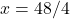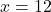Substituteinand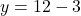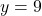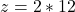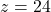This implies that;

Jaime is 12; Crystal is 9 and Marissa is 24 years old

Solving (d): Essence of the question

The question asks that we solve for the ages of Jaime, Crystal and Marissa